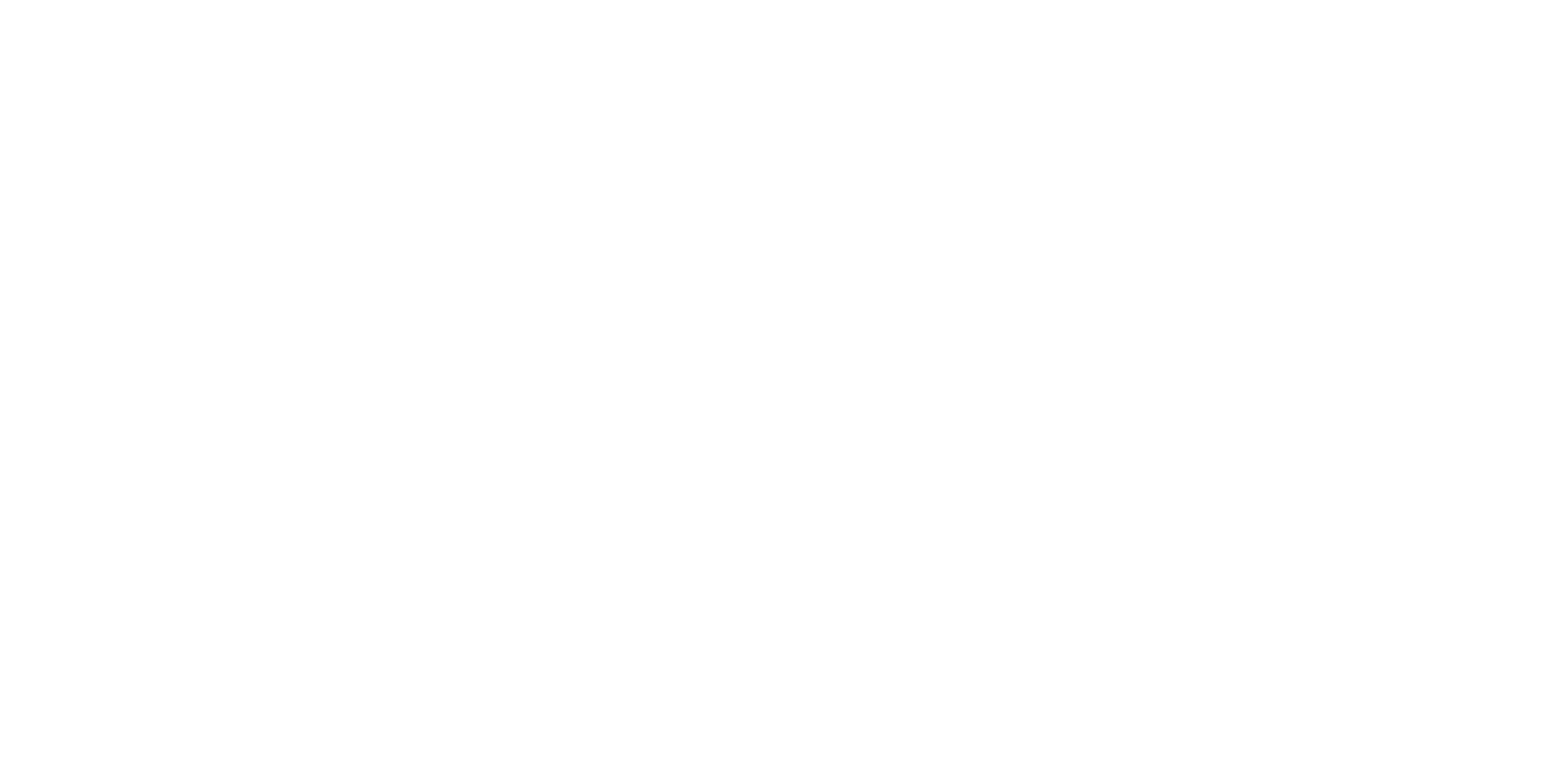Kuulchat

Online Education Resource Portal, its all freeWASSCE Nov 2018 mathematics

#### OBJECTIVE TEST

1.

Correct 9453 x 10-6 to 3 significant figures.
A.
0.009
B.
0.00945
C.
0.00950
D.
0.010

2.

Arrange the following in ascending order of magnitude: 0.45,¾ and 25%

A.

¾, 0.45, 25%

B.

¾, 25%, 0.45

C.

0.45, 25%, ¾

D.

25%, 0.45, ¾

3.

If log102 = 0.3010 and log102y = 1.8060, find, correct to the nearest whole number, the value of y.

A.

7

B.

6

C.

5

D.

4

4.

Simplify: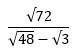A.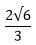B.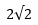C.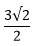D.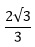5.

Which of the following describes the set P = {1,2,3,4,5,6}?

A.

P = {Prime numbers < 7}

B.

P = {x:x is a positive integer < 7}

C.

P = {rational numbers < 7}

D.

P = {x:x is a real number < 7}

6.

If y varies inversely as x and y = 6 when x = 3, find y when x = 9

A.

4

B.

3

C.

2

D.

1

7.

If the sequence x,4,16,y is Geometric Progression (GP), find the ratio of x:y.

A.

64:1

B.

8:1

C.

1:3

D.

1:64

8.

Make x the subject of the relation: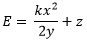A.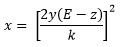B.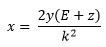C.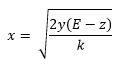D.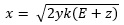9.

If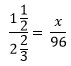, find the value of x.
A.

42

B.

45

C.

54

D.

64

10.

Find the equation whose roots are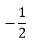and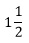.
A.

2x2 -4x + 6 = 0

B.

4x2 -4x - 3 = 0

C.

2x2 +3x + 4 = 0

D.

4x2 -4x + 3 = 0

11.

The graph is for the relation: y = d - x.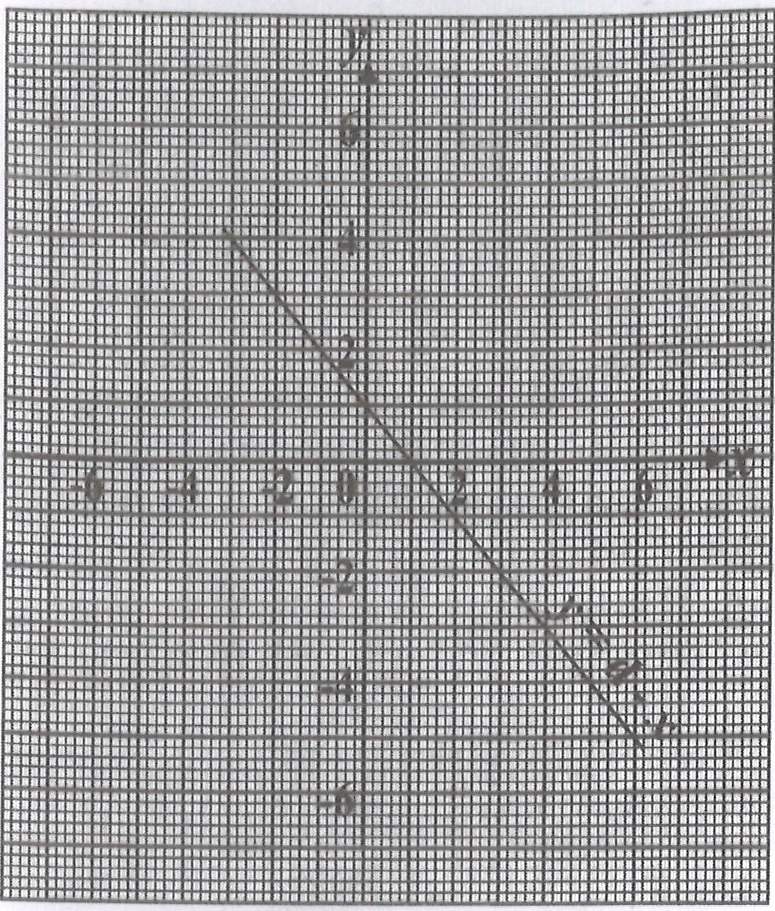Find the value of d.

A.

2

B.

1

C.

0

D.

-1

12.

Factorize: p2q2 - 6pqr + 9r2

A.

(pq-3r)2

B.

(pq - 3r)(pq + 3r)

C.

(pq+3r)2

D.

(pr+3q)(pr-3q)

13.

An empty rectangular tank is 250 cm long and 120 cm wide. If 180 litres of water is poured into the tank, calculate the height of the water.

A.

4.5 cm

B.

5.0 cm

C.

5.5 cm

D.

6.0 cm

14.

The area of a trapezium is 49 cm2. If the parallel sides are 6 cm and 7 cm long, find, correct to one decimal place, the distance between the parallel sides.

A.

6.5 cm

B.

6.8 cm

C.

7.4 cm

D.

7.5 cm

15.

The area of a sector of a circle and the length of its arc are 231 cm2 and 66 cm respectively. Calculate the radius of the circle

[Take Π = 22/7]

A.

3.5 cm

B.

7.0 cm

C.

10.5 cm

D.

14.0 cm

16.

A ladder, 10 m long, touches a side of a building at a height of 8 m. At what height would a ladder with length 12 m touch the building, if it makes the same angle with the ground?

(Assume that the ladder and building are on the same horizontal ground)

A.

10.6 m

B.

10.4 m

C.

10.0 m

D.

9.6 m